# NCERT Solutions for Class 8 Maths Chapter 11 Mensuration| PDF Download

On this page, you will get Chapter 11 Mensuration Class 8 Maths NCERT Solutions through which you will take advantage over your classmates and boost your preparation. Chapter 11 Mensuration NCERT Solutions PDF Download is also available here which will help you in developing problem solving skills. These Chapter 11 NCERT Solutions are accurate and detailed and as per the latest syllabus released by CBSE.

NCERT Solutions for Class 8 NCERT Solutions will improve the learning behaviour among students and increase their concentration. These NCERT Solutions will provide good experience and provide opportunities to learn new things.Page No: 171

Exercise 11.1

1. A square and a rectangular field with measurements as given in the figure have the same perimeter.Which field has a larger area?

Given:
The side of a square = 60 m and the length of rectangular field = 80 m
According to question,
Perimeter of rectangular file = Perimeter of square field
⇒ 2(l+b) = 4 × Side
⇒ 2(80 + b) = 4 × 60
⇒ (80 + b) = 240/2
⇒ (80 + b) = 120
⇒ b = 120 - 80
⇒ b = 40 m
Hence, the breadth of the rectangular field is 40 m.
Now,
Area of Square field= (Side)2
= (60)2 sq.m = 3600 sq.m
Area of Rectangular field = (length × breadth)
= 80 × 40 sq. m = 3200 sq. m
Hence, area of square field is larger.

2. Mrs. Kaushik has a square plot with the measurement as shown in the figure. She wants to construct a house in the middle of the plot. A garden is developed around the house. Find the total cost of developing a garden around the house at the rate of Rs. 55 per m2.Side of a square plot = 25 m
∴ Area of square plot = (Side)2 = (25)2 = 625 m2
Length and Breadth of the house is 20 m and 15 m respectively
∴ Area of the house = (length x breadth )
= 20 × 15 = 300 m2
Area of garden = Area of square plot – Area of house
= (625 – 300) = 325 m2
∵ Cost of developing the garden around the house is Rs.55
∴ Total Cost of developing the garden of area 325 sq. m = Rs.(55 × 325)
= Rs.17,875

2. The shape of a garden is rectangular in the middle and semi-circular at the ends as shown in the diagram. Find the area and the perimeter of this garden
[Length of rectangle is 20 – (3.5 + 3.5 meters]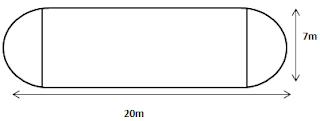Given:
Total length of the diagram = 20 m
Diameter of semi circle on both the ends = 7 m
∴ Radius of semi circle = diameter/ 2 = 7/2 = 3.5 m
Length of rectangular field = [Total length - (radius of semicircle on both side)]
={20 – (3.5 + 3.5)}
= 20 – 7 = 13 m
Breadth of the rectangular field = 7 m
∴ Area of rectangular field = ( l x b)
= (13×7) ⇒ 91 m2
Area of two semi circles = 2 × 1/2 πr2
= 2 × 1/2 × 22/7 × 3.5 × 3.5 = 38.5 m2
Total Area of garden = (91 + 38.5)⇒129.5 m2
Perimeter of two semi circles = 2 × πr = 2 × 22/7 × 3.5 = 22 m
Hence, Perimeter of garden = (22 + 13 + 13)m = 48 m

3. A flooring tile has the shape of a parallelogram whose base is 24 cm and the corresponding height is 10 cm. How many such tiles are required to cover a floor of area 1080 m2 ? [If required you can split the tiles in whatever way you want to fill up the corners]

Base of flooring tile = 24 cm ⇒ 0.24 m
height of a flooring tile = 10 cm
⇒ 0.10 m [1cm = 1/100 m]
Now, Area of flooring tile= Base × Altitude
= 0.24 × 0.10 sq. m
= 0.024 m2
∴ Number of tiles required to cover the floor = Area of floor/area of one tile
= 1080/0.024
= 45000 tiles
Hence 45000 tiles are required to cover the floor.

4. An ant is moving around a few food pieces of different shapes scattered on the floor. For which food-piece would the ant have to take a longer round? Remember, circumference of a circle can be obtained by using the expression c = 2πr, where r is the radius of the circle.
(a)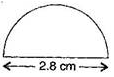(b)(c)(a) Radius = Diameter/2 = 2.8/2 = 1.4 cm
Circumference of semi circle = πr = 22/7 × 1.4 = 4.4 cmTotal distance covered by the ant= (Circumference of semi circle + Diameter)
=( 4.4 + 2.8 )cm = 7.2 cm

(b) Diameter of semi circle = 2.8 cmRadius = Diameter/2 = 2.8/2 = 1.4cm
Circumference of semi circle = πr = 22/7 × 1.4 = 4.4 cm
Total distance covered by the ant = (1.5 + 2.8 + 1.5 + 4.4) 10.2 cm

(c) Diameter of semi circle = 2.8 cmRadius = Diameter/2 = 2.8/2 = 1.4 cm
Circumference of semi circle = πr = 22/7 × 1.4 = 4.4 cm
Total distance covered by the ant= (2 + 2 + 4.4) = 8.4 cm
Hence for figure (b) food piece, the ant would take a longer round.

Page No. 177

Exercise 11.2

1. The shape of the top surface of a table is a trapezium. Find its area if its parallel sides are 1 m and 1.2 m and perpendicular distance between them is 0.8 m.Parallel side of the trapezium AB =1m , CD = 1.2 m and height (h) of the trapezium (AM) = 0.8 m
Area of top surface of the table = 1/2 (sum of parallel sides) Height
= 1/2 × (AB + CD) × AM
= 1/2 × ( 1 + 1.2) × 0.8
= 1/2 × 2.2 × 0.8
= 0.88 m2
Thus surface area of the table is 0.88 m2

2. The area of a trapezium is 34 cm2 and the length of one of the parallel sides is 10 cm and its height is 4 cm.Find the length of the other parallel side.

Let the length of the other parallel side be = b cm
Length of one parallel side = 10 am and height (h) = 4 cm
Area of trapezium = 1/2 (sum of parallel sides) Height
⇒ 34 = 1/2 ( a + b)h
⇒ 34 = 1/2 (10 + b) × 4
⇒ 34 = ( 10 + b) × 2
⇒ 34 = 20 + 2b
⇒ 34 - 20 = 2b
⇒ 14 = 2b
⇒ 7 = b
⇒ b = 7
Hence another required parallel side is 7 cm.

3. Length of the fence of a trapezium shaped field ABCD is 120 m. If BC = 48 m, CD = 17 m and AD = 40 m, find the area of this field. Side AB is perpendicular to the parallel sides AD and BC.Given: BC = 48 m, CD = 17 m,
AD = 40 m and perimeter = 120 m
∵ Perimeter of trapezium ABCD = Sum of all sides
120 =(AB + BC + CD + DA)
120 = AB + 48 + 17 + 40
120 = AB + 105
(120 – 105) = AB
AB = 15 m
Now Area of the field = 1/2 × ( Sum of parallel sides) × Height
= 1/2 × (BC + AD) × AB
= 1/2 × (48 + 40) × 15 m2
= 1/2 ×(88) × 15 m2
= 1/2 × (1320) m2
= 660 m2
Hence area of the field ABCD is 660  m2

4. The diagonal of a quadrilateral shaped field is 24 m and the perpendiculars dropped on it from the remaining opposite vertices are 8 m and 13 m. Find the area of the field.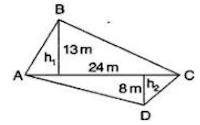Here h1 = 13 m, h2 = 8 m and AC = 24 m
Area of quadrilateral ABCD = Area of ABC + Area of ADC
= 1/2 b × h1+ 1/2 b × h2
= 1/2 b ( h1 + h2)
= 1/2 × 24 ( 13 + 8) m2
= 1/2 × 24 (21) m2
= 12 × 21 m2
= 252 m2
Hence required area of the field is 252  m2

5. The diagonals of a rhombus are 7.5 cm and 12 cm. Find its area.

Given: d1 =7.5 cm and d2 = 12 cm
Area of rhombus =12×(Product of digonals)
= 12 × (d1 × d2)
= 12×(7.5 × 12) cm2
= 45 cm2
Hence area of rhombus is 45 cm2

6. Find the area of a rhombus whose side is 6 cm and whose altitude is 4 cm. If one of the diagonals is 8 cm long, find the length of the other diagonal.

Rhombus is also a kind of Parallelogram.
∴ Area of rhombus= Base × Altitude
= (6 × 4) cm2
= 24 cm2
Also Area of rhombus = 12× (d1× d2)
⇒ 24 = 1/2 ×( 8 × d2)
⇒ 24 = 4 d2
⇒ 244 cm = d2
⇒ d2 = 6 cm
Hence, the length of the other diagonal is 6 cm.

7. The floor of a building consists of 3000 tiles which are rhombus shaped and each of its diagonals are 45 cm and 30 cm in length. Find the total cost of polishing the floor, if the cost per m2 is ₹4.

Here, d1 = 45 cm and d2 = 30 cm
∵ Area of one tile
= 12×(d1× d1)
= 12×(45 × 30 )
= 12×(350)
= 675 cm2
So, the area of one tile is 675 cm2

Area of 3000 tiles = 675 × 3000 cm2
= 2025000 cm2
= 2025000100∗100 m2
[1 cm = 1/100 m, Here cm2 = cm×cm = 1/100×1/100 m2]
= 202.50 m2
∵ Cost of polishing the floor per sq. meter = Rs. 4
∴ Cost of polishing the floor per 202.50 sq. meter = Rs. 4 × 202.50 = Rs. 810
Hence the total cost of polishing the floor is Rs. 810.

8. Mohan wants to buy a trapezium shaped field. Its side along the river is parallel to and twice the sidealong the road. If the area of this field is 10500 m2 and the perpendicular distance between the two parallel sides is 100 m, find the length of the side along the river.Given: Perpendicular distance (h) AM = 100 m
Area of the trapezium shaped field = 10500 m2
Let side along the road AB= x m
side along the river CD = 2x m
∴ Area of the trapezium field = 12× (AB + CD) x AM
10500 = 1/2 ( x + 2x) × 100
10500 = 3x × 50
3x = 10500/50
x = 10500/(50 × 3)
x = 70 m
Hence the side along the river = 2m = (2×70) = 140 m.

9. Top surface of a raised platform is in the shape of a regular octagon as shown in the figure. Find the area of the octagonal surface.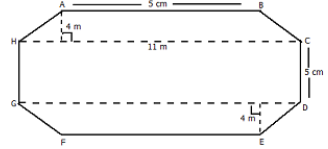Given: Octagon having eight equal sides, each 5 m.
Construction: Join HC and GD It will divide the octagon into two equal trapezium.
And AM is perpendicular on HC and EN is perpendicular on GD
Area of trap. ABCD = Area of trap. GDFE ---(1)
Area of two trapeziums = (area of trap. ABCH + area of trap. GDFE)
= (area of trap. ABCH + area of trap. ABCH) (by statement 1).
= (2×area of trap. ABCH )
= (2×1/2×(sum of parallel sides×height)
= (2×1/2×(AB + CH) x AM)
=(11 + 5)×4 m2
=(16) × 4
= 64 m2

Area of rectangle (HCDG ) = length × breadth
= HC×HG = 11×5 = 55 m2

Total area of octagon = Area of 2 Trapezium + Area of Rectangle
= 64 m2 + 55 m2 = 119 m2

10. There is a pentagonal shaped park as shown in the figure. For finding its are a Jyoti and Kavita divided it in two different ways.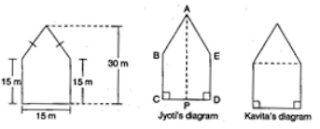Find the area of this park using both ways. Can you suggest some other way of finding its area?

First way: By Jyoti’s diagram,
Area of pentagon = Area of trapezium ABCP + Area of trapezium AEDP
= 1/2 (AP + BC) x CP + 1/2 (ED + AP) × DP
= 1/2 (30 + 15 ) x CP + 1/2(15 + 30) × DP
= 1/2 (30 + 15) (CP + DP)
= 1/2 × 45 × CD
= 1/2 × 45 × 15
= 337.5 m2
Second way: By Kavita’s diagram
Here, a perpendicular AM drawn to BE. AM = 30 – 15 = 15 m
Area of pentagon = Area of ABE + Area of square BCDE={12×15×15}+(15 × 15) m2
= (112.5 + 225.0) m2
= 337.5 m2
Hence total area of pentagon shaped park = 337.5 m2

11. Diagram of the adjacent picture frame has outer dimensions = 24 cm × 28 cm and inner dimensions 16 cm × 20 cm. Find the area of each section of theframe, if the width of each section is same.Here two of given figures (I) and (II) are similar in dimensions. And also figures (III) and (IV) are similar in dimensions.
Area of figure (I) = Area of trapezium
= 1/2 (a+b) × h = 1/2 (28 + 20) × 4
= 1/2 × 48 × 4 = 96 cm2
Also, Area of figure (II) = 96 cm2
Now Area of figure (III)
Area of trapezium = 1/2 (a + b) × h
= 1/2 (24 + 16) × 4
= 1/2 × 40 × 4
= 80 cm2
Also Area of figure (IV) = 80 cm2

Page No. 186

Exercise 11.3

1. There are two cuboidal boxes as shown in the adjoining figure. Which box requires the lesser amount of material to make?(a) Length of cuboidal box (l)= 60 cm
Breadth of cuboidal box (b) = 40 cm
Height of cuboidal box (h) = 50 cm
∴ Total surface area of cuboidal box = 2(lb+bh+hl)
= 2 (60 × 40 + 40 × 50 + 50 × 60) cm2
= 2 (2400 + 2000 + 3000) cm2
= 2 × 7400 cm2
= 14800 cm2

(b) Length of the cube is 50 cm
∴ Total surface area of cuboidal box =6(side)2
= 6 (50)2 cm2
= 6 (2500) cm2
= 15000 cm2
Thus, the cuboidal box (a) requires the lesser amount of material.

2. A suitcase with measures 80 cm × 48 cm × 24 cm is to be covered with a tarpaulin cloth. How many meters of tarpaulin of width 96 cm is required to cover 100 such suitcases?

Given:
Length of suitcase box (l) = 80 cm, Breadth of suitcase box (b) = 48 cm
And Height of cuboidal box (h) = 24 cm
∴ Total surface area of suitcase box= 2(lb+bh+hl)
= 2 (80 × 48 + 48 × 24 + 24 × 80) cm2
= 2 (3840 + 1152 + 1920)
= 2 × 6912 = 13824 cm2
Area of Tarpaulin cloth = Surface area of suitcase
⇒ l × b = 13824
⇒ l × 96 = 13824
⇒ l = 13824/96
= 144 cm
Required tarpaulin for 100 suitcases = (144 × 100) cm
= 14400 cm
= 144 m [ 1cm = 100 m]
Thus, 144 m tarpaulin cloth required to cover 100 suitcases.

3.Find the side of a cube whose surface area id 600 cm2

Here, Surface area of cube = 600 cm2
⇒ 6l2 = 600 cm2
⇒ l2 = 100 cm2
⇒ l = √100 cm
⇒ l = 10 cm
Hence the side of cube is 10 cm

4. Rukshar painted the outside of the cabinet of measure 1 m × 2 m × 1.5 m. How much surface area did she cover if she painted all except the bottom of the cabinet?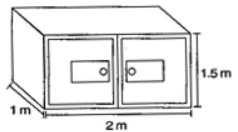Length of cabinet (l) = 2 m
Breadth of cabinet (b) = 1 m
Height of cabinet (h) = 1.5 m
∴ Surface area of cabinet = {Area of Base of cabinet (Cuboid) + Area of four walls}
= lb+2(l+b)h
={2×1 + 2(1+2) 1.5} m2
= 2 + 2 (3) 1.5 m2
= 2+6 (1.5) m2
= (2 + 9.0) m2
= 11 m2
Hence required surface area of cabinet is 11 m2

5. Daniel is paining the walls and ceiling of a cuboidal hall with length, breadth and height of 15 m, 10 m and 7 m respectively. From each can of paint 100 m2 of area is painted. How many cans of paint will she need to paint the room?

Length of wall (l) = 15 m
Breadth of wall (b) = 10 m
Height of wall (h) = 7 m
∴ Total Surface area of classroom=(Area of Base of ceiling (Cuboid) + Area of four walls)
= lb+2(l+b)h
= {15 × 10 + 2 (10 +15) (7)} m2
= {150 + 2 (25) (7)} m2
= (150 + 350) m2
= 500 m2
Area of one can is 100 m2
Now Required number of cans = Area of hall/ Area of one can = 500/100 = 5 cans
Hence 5 cans are required to paint the room.

6. Describe how the two figures below are alike and how they are different. Which box has larger lateral surface area?Diameter of cylinder = 7 cm
∴ Radius of cylinder (r) = 7/2 cm
Height of cylinder (h) = 7 cm
Lateral surface area of cylinder = 2πrh
= 2 × 22/7 × 7/2 × 7
= 154 cm2
Now lateral surface area of cube = 4(Side)= 4(7)2 cm2
= (4×49) cm2
= 196 cm2
Hence the cube has larger lateral surface area.

7.A closed cylindrical tank of radius 7 m and height 3 m is made from a sheet of metal. Howmuch sheet of metal is required?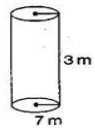Radius of cylindrical tank (r) = 7 m
Height of cylindrical tank (h) = 3 m
Total surface area of cylindrical tank = (Curved surface area + Area of upper end (circle)+ Area of Lower (circle) end)
= (2πrh+πr2+πr2)
= (2πrh+2πr2)
=2πr(h+r)
= 2 × 22/7 × 7(3 + 7) m2
= 44 × 10 m2
= 440 m2
Hence 440 m2 metal sheet is required.

8. The lateral surface area of a hollow cylinder is 4224 cm2. It is cut along its height and formed a rectangular sheet of width 33 cm. Find the perimeter of rectangular sheet?

Lateral surface area of hollow cylinder = 4224 cm2
Height of hollow cylinder = 33 cm
Curved surface area of hollow cylinder = 2πrh
⇒ 4224 = 2 × 22/7 × r × 33
⇒ r = (4224 × 7)/(2 × 22 × 33)
= (64 × 7)/22 cm
Now Length of rectangular sheet = 2πr
l = 2 × 22/7 × (64 × 7)/22
= 128 cm
Perimeter of rectangular sheet = 2(l + b)
= 2 (128 + 33)
= 2 × 161
= 322 cm
Hence perimeter of rectangular sheet is 322 cm.

9. A road roller takes 750 complete revolutions to move once over to level a road. Find the area of the road if the diameter of a road roller is 84 cm and length 1 m.Diameter of road roller = 84 cm
= 42 cm
Length of road roller (h) = 1 m = 100 cm
Curved surface area of road roller = 2πrh
= 2 × 22/7 × 42 × 100
= 26400 cm2
∴ Area covered by road roller in 750 revolutions = 26400 × 750 cm2
= 1,98,00,000 cm2
= 1980 m2 [∵ 1 m2= 10,000 cm2]
Thus, the area of the road is 1980 m2.

10. A company packages its milk powder in cylindrical container whose base has a diameter of 14 cm and height 20 cm. Company places a label around the surface of the container (as shown in figure). If the label is placed 2 cm from top and bottom, what is the area of the label?

Diameter of cylindrical container = 14 cm∴ Radius of cylindrical container (r) = d/2 = 14/2 = 7 cm
Height of cylindrical container = 20 cm
Height of the label (h) = (20 – 2 – 2) = 16 cm
Curved surface area of label = 2πrh
= 2×22/7×7×16
= 704 cm2
Hence the area of the label of 704 cm2.

Page No. 191

Exercise 11.4

1. Given a cylindrical tank, in which situation will you find surface area and in which situation volume.
(a) To find how much it can hold.
(b) Number of cement bags required to plaster it.
(c) To find the number of smaller tanks that can be filled with water from it.

(a) Volume (it is measure of the amount of space inside of a solild figures)
(b) Surface area (the outside part or uppermost layer of the soild figures)
(c) Volume

2. Diameter of cylinder A is 7 cm and the height is 14 cm. Diameter of cylinder B is 14 cm and height is 7 cm. Without doing any calculations can you suggest whose volume is greater? Verify it by finding the volume of both the cylinders. Check whether the cylinder with greater volume also has greater surface area.Yes, we can say that volume of cylinder B is greater, Because radius of cylinder B is greater than that of cylinder A.
Diameter of cylinder A = 7 cm
⇒ Radius(r) of cylinder A = 7/2 cm and Height(h) of cylinder A = 14 cm
∴ Volume of cylinder A = πr2h
= 22/7 × 7/2 ×7/2 × 14
= 539 cm3
Now Diameter of cylinder B = 14 cm
⇒ Radius of cylinder B = 14/2 = 7 cm and Height of cylinder B = 7 cm
∴ Volume of cylinder A = πr2h
= 22/7 × 7 ×7 ×7 cm3
= 1078 cm3

Since the cylinder A and cylinder B is open from upper end then it will exclude from the Total surface area

Total surface area of cylinder A = ( Area of lower end circle + curved surface area of cyliner)
= πr2 + 2πrh)
= πr(r+2h)
= (22/7 × 7/2) (7/2 + 2×14)
= 11(7/2+28)
= 11(31.5) cm2 = 346.5 cm2
Total surface area of cylinder B = πr(2h + r)
= 22/7 × 7(2×7 + 7)
= 22 × (14 + 7)
= 22 × 21 = 462 cm2
Yes, cylinder with greater volume also has greater surface area.

3. Find the height of a cuboid whose base area is 180 cm2 and volume is 900 cm3?

Let the Length, breadth and height of the cuboid be l, b, h.
Base of the cuboid is form a Recatangle so,that the Base(Reactangle) Area is (Length x Breadth)
Base area of cuboid = 180 cm2
L x B = 180 cm2 --- (1)
Volume of cuboid = l ×b × h
Volume of cuboid = 900 cm2
(lb)h = 900 (From eq. 1)
(180) h = 900
h = 900/180
= 5 m
Hence the height of cuboid is 5 m.

4. A cuboid is of dimensions 60 cm × 54 cm × 30 cm. How many small cubes with side 6 cm can be placed in the given cuboid?

Given: Length of cuboid (l) = 60 cm,
Breadth of cuboid (b) = 54 cm and
Height of cuboid (h) = 30 cm
We know that, Volume of cuboid = l × b ×h
= (60 × 54 × 30) cm3
And Volume of cube = (Side)3
= 6 × 6 × 6 cm3
∴ Number of small cubes = (Volume of cuboid)/( Volume of cube)
= (60×54×30)/(6×6× 6)
= 450
Hence required number of small cubes are 450.

5. Find the height of the cylinder whose volume if 1.54 m3 and diameter of the base is 140 cm.

Given: Volume of cylinder = 1.54 m3 and Diameter of cylinder = 140 cm
∴ Radius (r) = d/2 = 140/2 = 70 cm
= 70/100 m = 0.7m [1cm=1/100m]
Volume of cylinder = πr2h
⇒ 1.54 = 22/7 × 0.7 × 0.7 × h
⇒ h = (1.54 × 7)/(22 × 0.7 × 0.7)
⇒ h = ( 154 × 7 × 10 × 10)/( 22 × 7 × 7 × 100)
= 1 m
Hence height of the cylinder is 1 m.

6. A milk tank is in the form of cylinder whose radius is 1.5 m and length is 7 m. Find the quantity of milk in liters that can be stored in the tank.

Given: Radius of cylindrical tank (r) = 1.5 m
Height of cylindrical tank (h) = 7 mVolume of cylindrical tank = πr2h
= 22/7 × 1.5 × 1.5 × 7
= 49.5 m3
= 49.5 × 1000 liters [∵1m3 = 1000 liters]
= 49500 liters
Hence required quantity of milk is 49500 liters that can be stored in the tank.

7. If each edge of a cube is doubled,
(i) how many times will its surface area increase?
(ii) how many times will its volume increase?

Let, l units be the edge of the cube.
Surface area = 6l2
and Volume of the cube =  l3
When its edge is doubled = 2l

(i) 6(2l)2 = 6(4l2) = 4(6l2)
= 4 (Surface area) {∵6l= Surface area of the cube}
The surface area of the new cube will be 4 times that of the original cube.

(ii) Volume of cube (V) = l3
When edge of cube is doubled = 2l, then
Volume of new cube = (2l)3 = 8l3
Volume of new cube = 8× Volume of cube
Hence, volume will increase 8 times.

8. Water is pouring into a cuboidal reservoir at the rate of 60 liters per minute. If the volume of reservoir is 108m3, find the number of hours it will take to fill the reservoir.

Volume of reservoir = 108m3
= 108×1000 litres [1m3=1000 l]
=108000 litres
Since water is pouring into reservoir @ 60 litres per minute and in
Time taken to fill the reservoir = 108000/(60×60) hours
= 30 hours
Hence, 30 hours it will take to fill the reservoir.

## NCERT Solutions for Class 8 Maths Chapter 11 Mensuration

In Chapter 11 Mensuration Maths NCERT Solutions we are dealing with problems related to perimeter and area of other plane closed figures like quadrilaterals. We will also learn about surface area and volume of solids such as cube, cuboid and cylinder.

• A square, a rectangle, a trapezium, a rhombus, a parallelogram, a triangle, a circle, etc., are plane figures and the surfaces enclosed by their boundaries are called areas.

• The area of a square = Side × Side
• The area of a rectangle = Length × Breadth
• The area of a circle = πr2   [where r is the radius]
• The area of triangle = 1/2 × Base × Altitude
• The area of a parallelogram = Base × Height

Below you will find exercisewise NCERT Solutions of Chapter 11 Class 8 Maths that is very useful in building basic knowledge and concepts given in the chapter. You will get to learn about a lot of topics which you'll find interesting.

Here you will get accurate and detailed NCERT Solutions of Class 8 Maths prepared by Studyrankers experts that will be useful in scoring great marks in the exams and completing your homework on time.

### NCERT Solutions for Class 8 Maths Chapters:

 Chapter 1 Rational Numbers Chapter 2 Linear Equations in Variable Chapter 3 Understanding Quadrilaterals Chapter 4 Practical Geometry Chapter 5 Data Handling Chapter 6 Squares and Square Roots Chapter 7 Cubes and Cube Roots Chapter 8 Comparing Quantities Chapter 9 Algebraic Expressions and Identities Chapter 10 Visualising Solid Shapes Chapter 12 Exponents and Powers Chapter 13 Direct and Inverse Proportions Chapter 14 Factorization Chapter 15 Introduction to Graphs Chapter 16 Playing with Numbers

FAQ on Chapter 11 Mensuration

#### How many exercises in Chapter 11 Mensuration Class 8 Maths?

There are four exercises in Chapter 11 Mensuration NCERT Solutions which will be helpful in understanding the basic concepts embedded in the questions. These solutions can help you in solving supplementary Maths Books.

#### If the lateral surface of the cylinder is 500 cm² and its height is 10 cm, then find the radius of its base.

The lateral surface area is A =2πrh. The curved surface area is A =  500 cm2 and its height is 10 cm, hence
A =2πrh
500 = 2 × 3.14 × r × 10
500 = 62.8r
r = 500/62.8
= 7.96
Therefore the radius of the cylinder is 7.96 cm.

#### A horse is tethered by a rope 10 m long at a point. Find the area of the region where it can graze (π = 3.14)

The area of the region the horse can graze is circular with a radius equal to the length of the rope.
Area of the circle is πr2
= 3.14 × 102
= 3.14 × 100
=314
Hence the area of the region the horse can graze is 314cm2.

#### What are right circular cylinders?

The cylinder which has congruent circular faces that are parallel to each other. The line segment joining the center of circular faces is perpendicular to the base. Such cylinders are known as right circular cylinders.